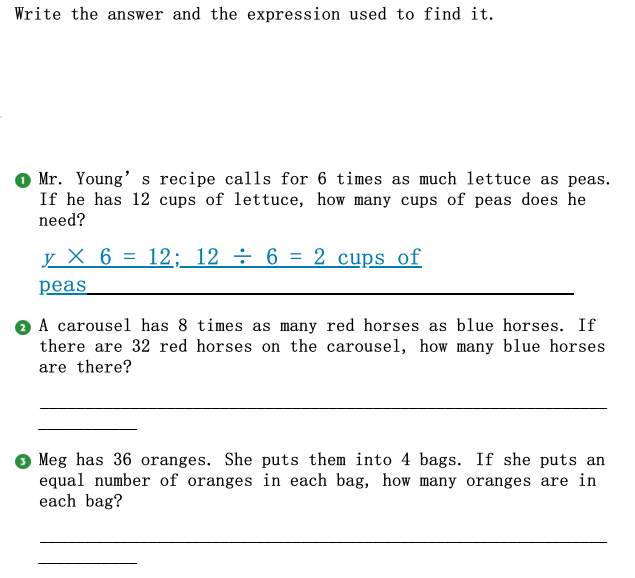### ¿Todavía tienes preguntas de matemáticas?

Pregunte a nuestros tutores expertos
Algebra
Pregunta(1) Mr. Young' s recipe calls for $$6$$ times as much lettuce as peas. If he has $$12$$ cups of lettuce, how many cups of peas does he need?

(2) A carousel has $$8$$ times as many red horses as blue horses. If there are $$32$$ red horses on the carousel, how many blue horses are there?

(3) Meg has $$36$$ oranges. She puts them into $$4$$ bags. If she puts an equal number of oranges in each bag, how many oranges are in each bag?